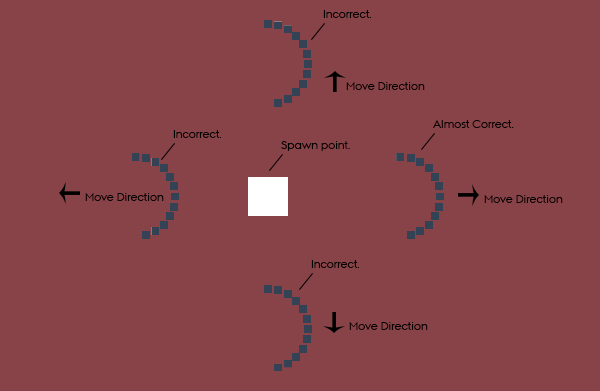# Bullet-Hell Tool (C#) - Rotate Half-Circle of Objects / Projectiles Towards Directional Vector2.

_Greetings
Greetings. I am working on c# script for a ‘bullet-hell’ tool and I have gotten stuck trying to produce a crescent / half-circle of objects - that turns toward the traveling direction. The scripts spawn a perfect half-circle every time and keep them there, no matter object-amount. No problem there.

_Question
What I am trying to achieve is for the half-circle to point towards the travel-direction of the centerObject. This travel direction is described via a Vector2 between (1,1) and (-1,-1). How can I use this vector to turn the half-circle in the direction of travel?

##There are multiple instances of InitializeOrbit, each responsible for its own half-circle and with a corresponding move direction Vector2.

_Code
In the first script, the ‘theta’ is first calculated, whereafter the objects are spawned.

``````public void InitializeOrbit()
{
for (int i = 0; i < numberOfOrbits; i++)
{
float theta = (1 * Mathf.PI / numberOfOrbits) * i;
Instantiate(OrbitPrefab, orbitLocation, transform.rotation);
}
}
``````

And in the second, the theta is used to calculate the position of the individual objects.

``````public void OrbitSource ()
{
transform.position = (Vector2)centerTransform.position + offset;
}
``````

I hope I have provided proper information, thanks in advance.

I managed to write a solution myself. I lot of information has been found in this stackoverflow which was a great help. Here’s the script:

``````public void InitializeOrbitProjectiles()
{
Vector3 posA = new Vector3(projectile.direction.y, -projectile.direction.x) + transform.position;
Vector3 posB = new Vector3(-projectile.direction.y, projectile.direction.x) + transform.position;

var centerDirection = Quaternion.LookRotation((posB - posA).normalized);
float directionAngle = Angle(projectile.direction);

for (int i = 0; i < projectile.numberOfOrbits; i++)
{
float angle = (projectile.orbitCircle * Mathf.PI / projectile.numberOfOrbits) * i;

float x = Mathf.Sin(angle) * projectile.orbitRadius;
float z = Mathf.Cos(angle) * projectile.orbitRadius;
Vector3 pos = new Vector3(0, 0, 0);

if ((directionAngle >= 0 && directionAngle < 90) || (directionAngle >= 271 && directionAngle < 360))
pos = new Vector3(0, x, z);
else if (directionAngle < 91)
pos = new Vector3(x, 0, z);
else if (directionAngle < 270)
pos = new Vector3(0, -x, z);
else
pos = new Vector3(-x, 0, z);

pos = centerDirection * pos;

Instantiate(projectileOrbitPrefab, transform.position + pos, transform.rotation);

}
}
``````

And the orbitSource Script:

``````public void OrbitSource()
{
transform.position = centerTransform.position + pos;
}
``````